# Water tanks

A different amount of water is poured into each of the three identical vessels. In the first vessel, water fills 30% of its volume and in the second vessel 40% of its volume. The third container contains 19 liters of water. If we distributed the water from all the vessels equally, the water in each vessel would fill two-fifths of its volume.

What is the volume of one container?

x =  38 l

### Step-by-step explanation:

0.30x + 0.40x+19 = 2/5•3•x

0.30•x + 0.40•x+19 = 2/5•3•x

2.5x = 95

x = 38

Our simple equation calculator calculates it.Did you find an error or inaccuracy? Feel free to write us. Thank you!Tips to related online calculators
Do you have a linear equation or system of equations and looking for its solution? Or do you have a quadratic equation?
Tip: Our volume units converter will help you with the conversion of volume units.

## Related math problems and questions:

• Bath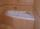In the bath is 30 liters of hot water. Then added 36 liters of cold water at temperature of 19 °C decreased temperature of water to 41 °C. What was the initial temperature of the hot water?
• PharmacistThe pharmacist had a 12 l vessel herbal lotion, which contained a 30% active ingredient. Her assistant poured 18 liters of herbal lotion containing 45% of the active ingredient into a container. What percentage of herbal lotion was produced?
• MilkThere were 22 liters of milk in three containers. There was 6 liters more in the first container than in the second. After pouring 5 liters from the first container into the third container, the same quantity of milk is in the second and third container.
• PharmacyAt the pharmacy are in one container 20% solution in the second 50% solution of disinfectant. They need to prepare 4 L of 48-percent solution. What amount of solution from each container is needed to mix?
• Four numbersThe first number is 50% second, the second number is 40% third, the third number is 20% of the fourth. The sum is 396. What are the numbers?
• 40% volume40% volume with 104 uph (units per labor hour) 8 people working. What is the volume?
• CompetitorsIn the first round of slalom fell 15% of all competitors and in the second round another ten racers. Together, 40% of all competitors fell. What was the total number of competitors?
• Lions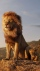If 8 lions is 40% how many would 75% be?
• SolutionWhat amount of 80% solution and what amount of water is needed to mix in order to obtain two liters of 60% solution of this mixture?
• Two water containers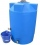In the first container, there are 200 m3 of water and in the second 40 m3. The first container will flow down at a rate of 10 m3 water per hour. At the same time flows to the second rate of 5 m3 per hour. After how many hours there will be three times les
• Two workers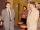Two workers make 138 parts/items. The first one produces 30% more than the second. How many items will each produce?
• Distilled waterSea water contains 5% salt. How many distilled water should be pour to 40 kg of sea water so that the salt content is 2%. How many kilograms of 2% of sea water we get?
• Two shopsIn two different shops, the same skis had the same price. In the first, however, they first became more expensive by 20% and then cheaper by 5%. In the second, they were first cheaper by 5% and then more expensive by 30%, so after these adjustments in the
• Pumps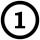First pump flows 16 liters per second into the basin by the second pump 75% of the first and by third pump half more than the second. How long will take fill basin by all three pumps simultaneously volume 15 m3 (cubic meters)?
• SolutionsHow much 60% solution and how much 35% solution is needed to create 100 l of 40% solution?
• Three piles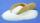100 kg of sugar we divide into three piles. The first pile is small. If we added to second 2 kg of sugar it would have 25% more sugar than the first pile. If we added to third pile 3 kg of sugar it would have 20% more sugar than the 2nd pile. How many kil
• Brunette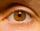Of the girls who attend primary school are 30% blondes and 70% brunette. 81% blondes and 19% brunette has blue eyes. How many girls attending school altogether if 470 girls has blue eyes?